﻿ 渗流力学边界条件的数理表达——聚焦于弹性波传播问题

# 渗流力学边界条件的数理表达——聚焦于弹性波传播问题Mathematical Expressions on the Boundary Conditions for Permeable Mechanics—Focusing on the Elastic Propagation Issue

Abstract: Cracks can be expressed as boundary conditions for permeable mechanics in the mathematical method. For the elastic wave propagation in a scenario with cracks, the boundary conditions for permeable mechanics on the cracks can be set as zero normal displacement, which is more con-sistent with the actual permeable phenomenon. By solving such mathematical expressions, one cannot only vividly describe the penetrating effect of elastic wave propagation across the cracks, but also effectively reflect the joint influence of factors including the length, the location and the exist-ence of endpoints of cracks on the elastic wave propagation.

1. 引言

2. 理论方法与基本假设

2.1. 带有完全匹配层的弹性波方程的等效积分弱形式

$\left\{\begin{array}{l}{\int }_{L}\left({s}_{y}{\tau }_{xx}\stackrel{⇀}{n}\text{δ}u+{s}_{x}{\tau }_{xy}\stackrel{⇀}{n}\text{δ}v\right)\text{d}L-{\iint }_{S}\left({s}_{y}{\tau }_{xx}\frac{\partial \text{δ}u}{\partial x}+{s}_{x}{\tau }_{xy}\frac{\partial \text{δ}v}{\partial y}\right)\text{d}S+{\iint }_{S}{s}_{x}{s}_{y}\rho {\omega }^{2}u\text{δ}u\text{d}S=0\\ {\int }_{L}\left({s}_{y}{\tau }_{xy}\stackrel{⇀}{n}\text{δ}u+{s}_{x}{\tau }_{yy}\stackrel{⇀}{n}\text{δ}v\right)\text{d}L-{\iint }_{S}\left({s}_{y}{\tau }_{xy}\frac{\partial \text{δ}v}{\partial x}+{s}_{x}{\tau }_{yy}\frac{\partial \text{δ}u}{\partial y}\right)\text{d}S+{\iint }_{S}{s}_{x}{s}_{y}\rho {\omega }^{2}v\text{δ}v\text{d}S=0\end{array}$ (1)

2.2. 对裂隙的基本假设

① 裂隙宽度趋于零；

② 裂隙存在与否对弹性波传播具有实质影响，而裂隙内填充的物质对弹性波传播的影响不大；

③ 弹性波传播到裂隙处时，部分或全部法向连续性边界条件丧失；

④ 为确保弹性波穿透裂隙，切向连续性边界条件不作处理。

2.3. 边界条件的数学表达

2.3.1. 弱渗流裂隙

① 法向位移为零、法向应力连续。此时有

$\stackrel{⇀}{n}\cdot {\left[\begin{array}{cc}{u}^{{L}_{0}}& {v}^{{L}_{0}}\end{array}\right]}^{\text{T}}=0$ (2)

② 法向位移连续、法向应力为零。此时有

$\stackrel{⇀}{n}\cdot {\tau }^{{L}_{0}}=0$ (3)

2.3.2. 强渗流裂隙

3. 应用

3.1. 裂隙对弹性波传播的影响

3.1.1. 模型与参数

① 弱渗流裂隙，法向位移为零、法向应力连续。此时根据式(2)，有

${u}^{{L}_{0}}=0$ (4)

② 弱渗流裂隙，法向位移连续、法向应力为零。此时根据式(3)，有

${\tau }_{xx}^{{L}_{0}}=0$ (5)

$\rho {v}_{p}^{2}\frac{\partial {v}_{x}^{{L}_{0}}}{\partial x}+\rho \left({v}_{p}^{2}-2{v}_{s}^{2}\right)\frac{\partial {v}_{z}^{{L}_{0}}}{\partial z}=0$ (6)

③ 强渗流裂隙，同时满足法向位移为零、法向应力为零。此时式(4)和式(6)共同成立。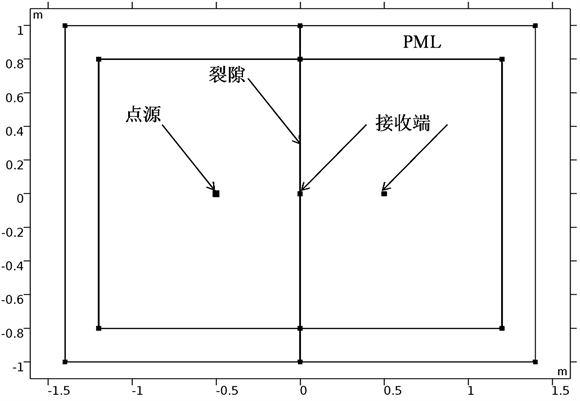Figure 1. No. 1 modelTable 1. Elastic parameters of a material

3.1.2. 结果与讨论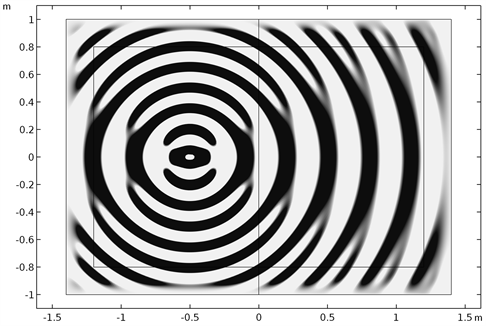(a)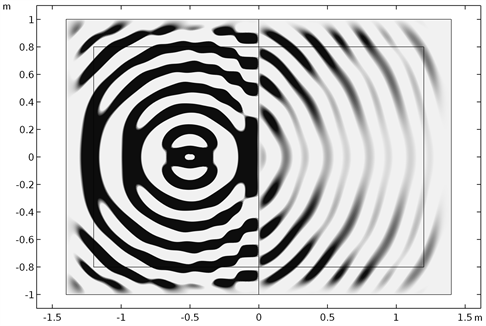(b)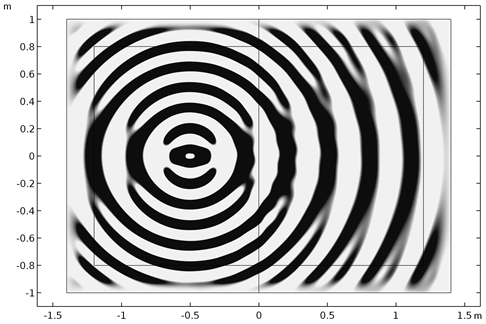(c)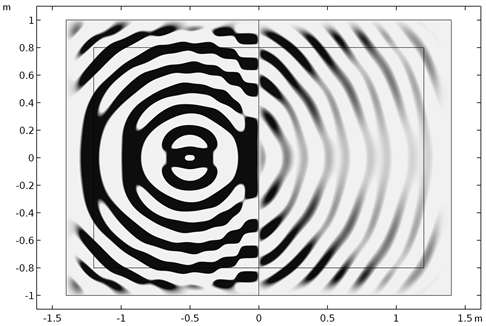(d)

Figure 2. Effect of a crack on the elastic propagation response with a frequency of 10 kHz (In the model). (a) Without a crack; (b) With a weak permeable crack of type ①; (c) With a weak permeable crack of type ②; (d) With an enhanced permeable crackTable 2. Effect of a crack on the elastic propagation response with a frequency of 10 kHz (At the receiver)

3.2. 裂隙端点对弹性波传播的影响

3.2.1. 模型与参数

${u}^{{L}_{0}}\mathrm{cos}\theta +{v}^{{L}_{0}}\mathrm{sin}\theta =0$ (7)

$\theta ={45}^{\circ }$ 时，式(7)简化为

${u}^{{L}_{0}}+{v}^{{L}_{0}}=0$ (8)

${\tau }_{{45}^{\circ }}^{{L}_{0}}={\tau }_{xx}^{{L}_{0}}{\mathrm{cos}}^{2}\theta +{\tau }_{yy}^{{L}_{0}}{\mathrm{sin}}^{2}\theta -2{\tau }_{xy}^{{L}_{0}}\mathrm{cos}\theta \mathrm{sin}\theta =0$ (9)

$\theta ={45}^{\circ }$ 时，式(9)简化为

${\tau }_{xx}^{{L}_{0}}+{\tau }_{yy}^{{L}_{0}}-2{\tau }_{xy}^{{L}_{0}}=0$ (10)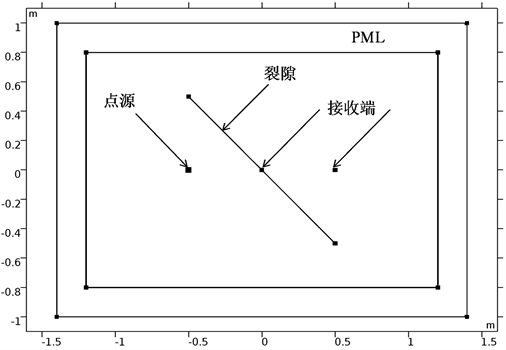Figure 3. No. 2 model

3.2.2. 结果与讨论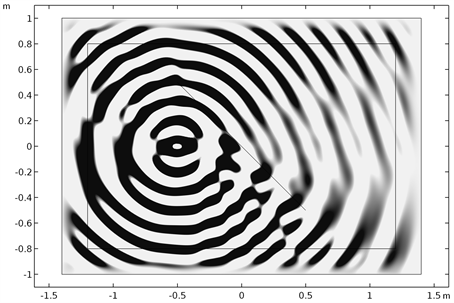(a)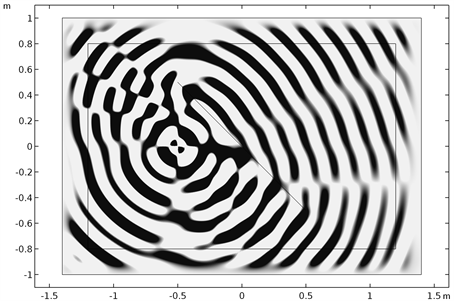(b)

Figure 4. Effect of crack end point on the elastic propagation response with a frequency of 10 kHz (In the model). (a) u component; (b) v componentTable 3. Effect of crack end point on the elastic propagation response with a frequency of 10 kHz (At the receiver and the end point)

4. 结论

(1) 将裂隙作为边界条件进行处理，不失为探究其渗流力学作用的有效策略；

(2) 对于弹性波传播问题，法向位移为零的渗流力学边界条件与实际现象更加符合；

(3) 裂隙处的法向位移为零，并不必然代表弹性波法向位移无法穿透裂隙传播。

 程林松. 高等渗流力学[M]. 北京: 石油工业出版社, 2011.

 张阔, 刘鹤. 增材制造技术中的弹性各向异性影响因素[J]. 工业技术创新, 2017, 4(4): 53-58.

 张阔. 增材制造技术中基于时域有限元方法的声波–弹性波耦合(一): 理论[J]. 工业技术创新, 2019, 6(5): 74-85, 90.

 张阔. 增材制造技术中基于时域有限元方法的声波–弹性波耦合(二): 应用[J]. 工业技术创新, 2019, 6(6): 75-86.

 张阔. 隧道效应下的Schrödinger方程吸收边界条件[J]. 新一代信息技术, 2019, 2(17): 47-52.

 张阔. 柱状模型传递矩阵校正图谱[J]. 新一代信息技术, 2020, 3(2): 1-9.

 张阔. 各向同性负泊松比超材料弹性参数线性化初探[J]. 工业技术创新, 2018, 5(4): 53-60.

 张阔. 关于半整数维波动方程求解的几点注记[J]. 新一代信息技术, 2019, 2(21): 13-21.

Top